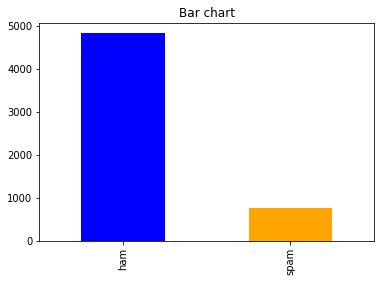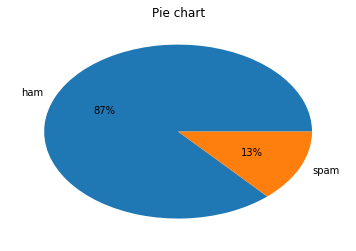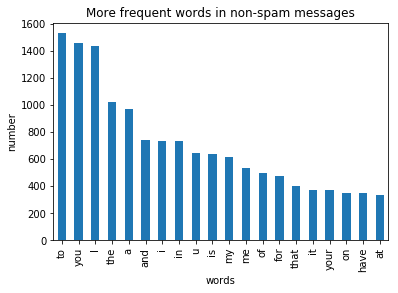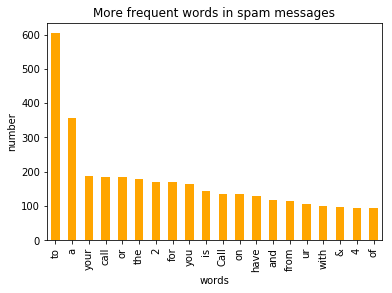In :
``import jovian``
In :
``````import numpy as np
import pandas as pd
import matplotlib.pyplot as plt
from collections import Counter
from sklearn import feature_extraction, model_selection, naive_bayes, metrics, svm
from IPython.display import Image
import warnings
warnings.filterwarnings("ignore")
%matplotlib inline
``````
In :
``````data=pd.read_csv(r"spam.csv",encoding='latin-1')
print(data.head(10))``````
``` v1 v2 Unnamed: 2 \ 0 ham Go until jurong point, crazy.. Available only ... NaN 1 ham Ok lar... Joking wif u oni... NaN 2 spam Free entry in 2 a wkly comp to win FA Cup fina... NaN 3 ham U dun say so early hor... U c already then say... NaN 4 ham Nah I don't think he goes to usf, he lives aro... NaN 5 spam FreeMsg Hey there darling it's been 3 week's n... NaN 6 ham Even my brother is not like to speak with me. ... NaN 7 ham As per your request 'Melle Melle (Oru Minnamin... NaN 8 spam WINNER!! As a valued network customer you have... NaN 9 spam Had your mobile 11 months or more? U R entitle... NaN Unnamed: 3 Unnamed: 4 0 NaN NaN 1 NaN NaN 2 NaN NaN 3 NaN NaN 4 NaN NaN 5 NaN NaN 6 NaN NaN 7 NaN NaN 8 NaN NaN 9 NaN NaN ```
In :
``````count_Class=pd.value_counts(data["v1"], sort= True)
count_Class.plot(kind= 'bar', color= ["blue", "orange"])
plt.title('Bar chart')
plt.show()
``````In :
``````count_Class.plot(kind = 'pie',  autopct='%1.0f%%')
plt.title('Pie chart')
plt.ylabel('')
plt.show()
``````In :
``````count1 = Counter(" ".join(data[data['v1']=='ham']["v2"]).split()).most_common(20)
df1 = pd.DataFrame.from_dict(count1)
df1 = df1.rename(columns={0: "words in non-spam", 1 : "count"})
count2 = Counter(" ".join(data[data['v1']=='spam']["v2"]).split()).most_common(20)
df2 = pd.DataFrame.from_dict(count2)
df2 = df2.rename(columns={0: "words in spam", 1 : "count_"})

``````
In :
``````df1.plot.bar(legend = False)
y_pos = np.arange(len(df1["words in non-spam"]))
plt.xticks(y_pos, df1["words in non-spam"])
plt.title('More frequent words in non-spam messages')
plt.xlabel('words')
plt.ylabel('number')
plt.show()

``````In :
``````df2.plot.bar(legend = False, color = 'orange')
y_pos = np.arange(len(df2["words in spam"]))
plt.xticks(y_pos, df2["words in spam"])
plt.title('More frequent words in spam messages')
plt.xlabel('words')
plt.ylabel('number')
plt.show()

``````In :
``````f = feature_extraction.text.CountVectorizer(stop_words = 'english')
X = f.fit_transform(data["v2"])
np.shape(X)
``````
Out:
``(5572, 8404)``
In :
``````data["v1"]=data["v1"].map({'spam':1,'ham':0})
print(data)
X_train, X_test, y_train, y_test = model_selection.train_test_split(X, data['v1'], test_size=0.33, random_state=42)
print([np.shape(X_train), np.shape(X_test)])
``````
``` v1 v2 Unnamed: 2 \ 0 NaN Go until jurong point, crazy.. Available only ... NaN 1 NaN Ok lar... Joking wif u oni... NaN 2 NaN Free entry in 2 a wkly comp to win FA Cup fina... NaN 3 NaN U dun say so early hor... U c already then say... NaN 4 NaN Nah I don't think he goes to usf, he lives aro... NaN 5 NaN FreeMsg Hey there darling it's been 3 week's n... NaN 6 NaN Even my brother is not like to speak with me. ... NaN 7 NaN As per your request 'Melle Melle (Oru Minnamin... NaN 8 NaN WINNER!! As a valued network customer you have... NaN 9 NaN Had your mobile 11 months or more? U R entitle... NaN 10 NaN I'm gonna be home soon and i don't want to tal... NaN 11 NaN SIX chances to win CASH! From 100 to 20,000 po... NaN 12 NaN URGENT! You have won a 1 week FREE membership ... NaN 13 NaN I've been searching for the right words to tha... NaN 14 NaN I HAVE A DATE ON SUNDAY WITH WILL!! NaN 15 NaN XXXMobileMovieClub: To use your credit, click ... NaN 16 NaN Oh k...i'm watching here:) NaN 17 NaN Eh u remember how 2 spell his name... Yes i di... NaN 18 NaN Fine if thatåÕs the way u feel. ThatåÕs the wa... NaN 19 NaN England v Macedonia - dont miss the goals/team... NaN 20 NaN Is that seriously how you spell his name? NaN 21 NaN IÛ÷m going to try for 2 months ha ha only joking NaN 22 NaN So Ì_ pay first lar... Then when is da stock c... NaN 23 NaN Aft i finish my lunch then i go str down lor. ... NaN 24 NaN Ffffffffff. Alright no way I can meet up with ... NaN 25 NaN Just forced myself to eat a slice. I'm really ... NaN 26 NaN Lol your always so convincing. NaN 27 NaN Did you catch the bus ? Are you frying an egg ... NaN 28 NaN I'm back &amp; we're packing the car now, I'll... NaN 29 NaN Ahhh. Work. I vaguely remember that! What does... NaN ... .. ... ... 5542 NaN Armand says get your ass over to epsilon NaN 5543 NaN U still havent got urself a jacket ah? NaN 5544 NaN I'm taking derek &amp; taylor to walmart, if I... NaN 5545 NaN Hi its in durban are you still on this number NaN 5546 NaN Ic. There are a lotta childporn cars then. NaN 5547 NaN Had your contract mobile 11 Mnths? Latest Moto... NaN 5548 NaN No, I was trying it all weekend ;V NaN 5549 NaN You know, wot people wear. T shirts, jumpers, ... NaN 5550 NaN Cool, what time you think you can get here? NaN 5551 NaN Wen did you get so spiritual and deep. That's ... NaN 5552 NaN Have a safe trip to Nigeria. Wish you happines... NaN 5553 NaN Hahaha..use your brain dear NaN 5554 NaN Well keep in mind I've only got enough gas for... NaN 5555 NaN Yeh. Indians was nice. Tho it did kane me off ... NaN 5556 NaN Yes i have. So that's why u texted. Pshew...mi... NaN 5557 NaN No. I meant the calculation is the same. That ... NaN 5558 NaN Sorry, I'll call later NaN 5559 NaN if you aren't here in the next &lt;#&gt; hou... NaN 5560 NaN Anything lor. Juz both of us lor. NaN 5561 NaN Get me out of this dump heap. My mom decided t... NaN 5562 NaN Ok lor... Sony ericsson salesman... I ask shuh... NaN 5563 NaN Ard 6 like dat lor. NaN 5564 NaN Why don't you wait 'til at least wednesday to ... NaN 5565 NaN Huh y lei... NaN 5566 NaN REMINDER FROM O2: To get 2.50 pounds free call... NaN 5567 NaN This is the 2nd time we have tried 2 contact u... NaN 5568 NaN Will Ì_ b going to esplanade fr home? NaN 5569 NaN Pity, * was in mood for that. So...any other s... NaN 5570 NaN The guy did some bitching but I acted like i'd... NaN 5571 NaN Rofl. Its true to its name NaN Unnamed: 3 Unnamed: 4 0 NaN NaN 1 NaN NaN 2 NaN NaN 3 NaN NaN 4 NaN NaN 5 NaN NaN 6 NaN NaN 7 NaN NaN 8 NaN NaN 9 NaN NaN 10 NaN NaN 11 NaN NaN 12 NaN NaN 13 NaN NaN 14 NaN NaN 15 NaN NaN 16 NaN NaN 17 NaN NaN 18 NaN NaN 19 NaN NaN 20 NaN NaN 21 NaN NaN 22 NaN NaN 23 NaN NaN 24 NaN NaN 25 NaN NaN 26 NaN NaN 27 NaN NaN 28 NaN NaN 29 NaN NaN ... ... ... 5542 NaN NaN 5543 NaN NaN 5544 NaN NaN 5545 NaN NaN 5546 NaN NaN 5547 NaN NaN 5548 NaN NaN 5549 NaN NaN 5550 NaN NaN 5551 NaN NaN 5552 NaN NaN 5553 NaN NaN 5554 NaN NaN 5555 NaN NaN 5556 NaN NaN 5557 NaN NaN 5558 NaN NaN 5559 NaN NaN 5560 NaN NaN 5561 NaN NaN 5562 NaN NaN 5563 NaN NaN 5564 NaN NaN 5565 NaN NaN 5566 NaN NaN 5567 NaN NaN 5568 NaN NaN 5569 NaN NaN 5570 NaN NaN 5571 NaN NaN [5572 rows x 5 columns] [(3733, 8404), (1839, 8404)] ```
In :
``````#
list_alpha = np.arange(1/100000, 20, 0.11)
score_train = np.zeros(len(list_alpha))
score_test = np.zeros(len(list_alpha))
recall_test = np.zeros(len(list_alpha))
precision_test= np.zeros(len(list_alpha))
count = 0
for alpha in list_alpha:
bayes = naive_bayes.MultinomialNB(alpha=alpha)
bayes.fit(X_train, y_train)
score_train[count] = bayes.score(X_train, y_train)
score_test[count]= bayes.score(X_test, y_test)
recall_test[count] = metrics.recall_score(y_test, bayes.predict(X_test))
precision_test[count] = metrics.precision_score(y_test, bayes.predict(X_test))
count = count + 1 ``````
In :
``````matrix = np.matrix(np.c_[list_alpha, score_train, score_test, recall_test, precision_test])
models = pd.DataFrame(data = matrix, columns =
['alpha', 'Train Accuracy', 'Test Accuracy', 'Test Recall', 'Test Precision'])
models.head(n=10)``````
Out:
In :
``````best_index = models['Test Precision'].idxmax()
models.iloc[best_index, :]
``````
Out:
``````alpha             15.730010
Train Accuracy     0.979641
Test Accuracy      0.969549
Test Recall        0.777778
Test Precision     1.000000
Name: 143, dtype: float64``````
In :
``models[models['Test Precision']==1].head(n=5)``
Out:
In :
``````best_index = models[models['Test Precision']==1]['Test Accuracy'].idxmax()
bayes = naive_bayes.MultinomialNB(alpha=list_alpha[best_index])
bayes.fit(X_train, y_train)
models.iloc[best_index, :]
``````
Out:
``````alpha             15.730010
Train Accuracy     0.979641
Test Accuracy      0.969549
Test Recall        0.777778
Test Precision     1.000000
Name: 143, dtype: float64``````
In :
``````m_confusion_test = metrics.confusion_matrix(y_test, bayes.predict(X_test))
pd.DataFrame(data = m_confusion_test, columns = ['Predicted 0', 'Predicted 1'],
index = ['Actual 0', 'Actual 1'])``````
Out:
In :
``````list_C = np.arange(500, 2000, 100) #100000
score_train = np.zeros(len(list_C))
score_test = np.zeros(len(list_C))
recall_test = np.zeros(len(list_C))
precision_test= np.zeros(len(list_C))
count = 0
for C in list_C:
svc = svm.SVC(C=C)
svc.fit(X_train, y_train)
score_train[count] = svc.score(X_train, y_train)
score_test[count]= svc.score(X_test, y_test)
recall_test[count] = metrics.recall_score(y_test, svc.predict(X_test))
precision_test[count] = metrics.precision_score(y_test, svc.predict(X_test))
count = count + 1 ``````
In :
``````matrix = np.matrix(np.c_[list_C, score_train, score_test, recall_test, precision_test])
models = pd.DataFrame(data = matrix, columns =
['C', 'Train Accuracy', 'Test Accuracy', 'Test Recall', 'Test Precision'])
models.head(n=10)``````
Out:
In :
``````best_index = models['Test Precision'].idxmax()
models.iloc[best_index, :]
``````
Out:
``````C                 500.000000
Train Accuracy      0.994910
Test Accuracy       0.982599
Test Recall         0.873016
Test Precision      1.000000
Name: 0, dtype: float64``````
In :
``models[models['Test Precision']==1].head(n=5)``
Out:
In :
``````best_index = models[models['Test Precision']==1]['Test Accuracy'].idxmax()
svc = svm.SVC(C=list_C[best_index])
svc.fit(X_train, y_train)
models.iloc[best_index, :]
``````
Out:
``````C                 800.000000
Train Accuracy      0.997053
Test Accuracy       0.983143
Test Recall         0.876984
Test Precision      1.000000
Name: 3, dtype: float64``````
In :
``````m_confusion_test = metrics.confusion_matrix(y_test, svc.predict(X_test))
pd.DataFrame(data = m_confusion_test, columns = ['Predicted 0', 'Predicted 1'],
index = ['Actual 0', 'Actual 1'])``````
Out:
In :
``````from sklearn.feature_extraction import DictVectorizer
from keras.models import load_model

mytest=("Mobile no. has won 500000 ")
Y = [mytest]#mytest is a new email in string format
f = feature_extraction.text.CountVectorizer(stop_words = 'english')

f.fit(data["v2"]) # fittingf

X = f.transform(Y)
res=bayes.predict(X)
print(res)
``````
``` ```
In [ ]:
``jovian.commit(artifacts=['spam.csv'])``
```[jovian] Saving notebook.. ```
In [ ]:
`` ``